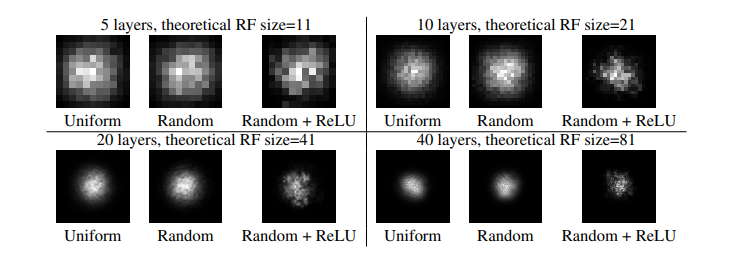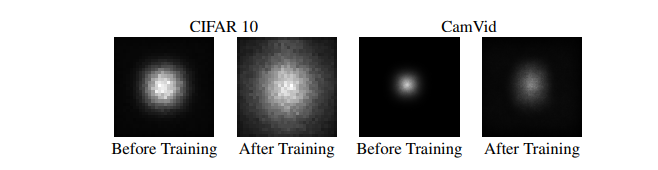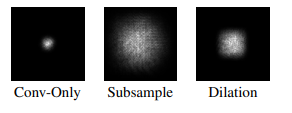# 论文解读《Understanding the Effective Receptive Field in Deep Convolutional Neural Networks》

empirical， 层数越深， 能够感知的patch的尺寸也越大，但是这样会付出更多的计算成本和时间消耗，所以需要通过traceback：

function receptive_field_sizes()

% compute input size from a given output size
f = @(output_size, ksize, stride) (output_size - 1) * stride + ksize;

%% n=1 discriminator

% fix the output size to 1 and derive the receptive field in the input
out = ...
f(f(f(1, 4, 1), ...   % conv2 -> conv3
4, 1), ...   % conv1 -> conv2
4, 2);       % input -> conv1

fprintf('n=1 discriminator receptive field size: %d\n', out);

%% n=2 discriminator

% fix the output size to 1 and derive the receptive field in the input
out = ...
f(f(f(f(1, 4, 1), ...   % conv3 -> conv4
4, 1), ...   % conv2 -> conv3
4, 2), ...   % conv1 -> conv2
4, 2);       % input -> conv1

fprintf('n=2 discriminator receptive field size: %d\n', out);

%% n=3 discriminator

% fix the output size to 1 and derive the receptive field in the input
out = ...
f(f(f(f(f(1, 4, 1), ...   % conv4 -> conv5
4, 1), ...   % conv3 -> conv4
4, 2), ...   % conv2 -> conv3
4, 2), ...   % conv1 -> conv2
4, 2);       % input -> conv1

fprintf('n=3 discriminator receptive field size: %d\n', out);

%% n=4 discriminator

% fix the output size to 1 and derive the receptive field in the input
out = ...
f(f(f(f(f(f(1, 4, 1), ...   % conv5 -> conv6
4, 1), ...   % conv4 -> conv5
4, 2), ...   % conv3 -> conv4
4, 2), ...   % conv2 -> conv3
4, 2), ...   % conv1 -> conv2
4, 2);       % input -> conv1

fprintf('n=4 discriminator receptive field size: %d\n', out);

%% n=5 discriminator

% fix the output size to 1 and derive the receptive field in the input
out = ...
f(f(f(f(f(f(f(1, 4, 1), ...   % conv6 -> conv7
4, 1), ...   % conv5 -> conv6
4, 2), ...   % conv4 -> conv5
4, 2), ...   % conv3 -> conv4
4, 2), ...   % conv2 -> conv3
4, 2), ...   % conv1 -> conv2
4, 2);       % input -> conv1

fprintf('n=5 discriminator receptive field size: %d\n', out);--如上图所示，在残差网络中不使用池化和下采样方法，随着训练的进行，有效感知野的范围在提升，同时即时感知野的大小已经大于了整个图像的整个大小，有效感知野的范围还是不能够覆盖整个图像。

--在残差网络架构的模型中，使用subsampling 技术，理论感知域增加的非常大，但是有效感知域也是十分小的（如上图的右边Cam所示，堆叠多个VGG）；有效减缓 有效感知域的高斯分布的方法带来的影响

-- 和直接搭建多层卷积网络相比，下采样和扩张卷积（ dilate conv）技术都可以增大有效感知野的大小，如下图所示：如何有效的对抗感知野的高斯分布呢？

-- 操纵权重的初始化 使卷积核中心的权值更小，外部的权值更大，优化 w 去最大化 有效感知野的大小。

-- 认为 从CNN的结构来看是一个很好的增大有效感知域的措施，比如 dilate conv，skip-connection 使得感知野更小了，dropout 并不会改变有效感知野

posted @ 2019-09-05 22:14  陈柯成  阅读(2397)  评论(0编辑  收藏  举报